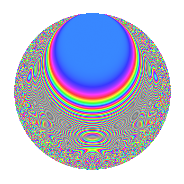Properties

 Label 1003.2.aLevel 1003 Weight 2 Character orbit a Rep. character $$\chi_{1003}(1,\cdot)$$ Character field $$\Q$$ Dimension 77 Newforms 10 Sturm bound 180 Trace bound 2

Related objects

Defining parameters

 Level: $$N$$ = $$1003 = 17 \cdot 59$$ Weight: $$k$$ = $$2$$ Character orbit: $$[\chi]$$ = 1003.a (trivial) Character field: $$\Q$$ Newforms: $$10$$ Sturm bound: $$180$$ Trace bound: $$2$$ Distinguishing $$T_p$$: $$2$$, $$3$$

Dimensions

The following table gives the dimensions of various subspaces of $$M_{2}(\Gamma_0(1003))$$.

Total New Old
Modular forms 92 77 15
Cusp forms 89 77 12
Eisenstein series 3 0 3

The following table gives the dimensions of the cuspidal new subspaces with specified eigenvalues for the Atkin-Lehner operators and the Fricke involution.

$$17$$$$59$$FrickeDim.
$$+$$$$+$$$$+$$$$18$$
$$+$$$$-$$$$-$$$$22$$
$$-$$$$+$$$$-$$$$20$$
$$-$$$$-$$$$+$$$$17$$
Plus space$$+$$$$35$$
Minus space$$-$$$$42$$

Trace form

 $$77q$$ $$\mathstrut -\mathstrut q^{2}$$ $$\mathstrut +\mathstrut 75q^{4}$$ $$\mathstrut +\mathstrut 2q^{5}$$ $$\mathstrut +\mathstrut 4q^{6}$$ $$\mathstrut -\mathstrut 8q^{7}$$ $$\mathstrut +\mathstrut 3q^{8}$$ $$\mathstrut +\mathstrut 77q^{9}$$ $$\mathstrut +\mathstrut O(q^{10})$$ $$77q$$ $$\mathstrut -\mathstrut q^{2}$$ $$\mathstrut +\mathstrut 75q^{4}$$ $$\mathstrut +\mathstrut 2q^{5}$$ $$\mathstrut +\mathstrut 4q^{6}$$ $$\mathstrut -\mathstrut 8q^{7}$$ $$\mathstrut +\mathstrut 3q^{8}$$ $$\mathstrut +\mathstrut 77q^{9}$$ $$\mathstrut +\mathstrut 18q^{10}$$ $$\mathstrut +\mathstrut 4q^{11}$$ $$\mathstrut +\mathstrut 16q^{12}$$ $$\mathstrut -\mathstrut 10q^{13}$$ $$\mathstrut +\mathstrut 12q^{14}$$ $$\mathstrut +\mathstrut 20q^{15}$$ $$\mathstrut +\mathstrut 67q^{16}$$ $$\mathstrut -\mathstrut 3q^{17}$$ $$\mathstrut -\mathstrut 17q^{18}$$ $$\mathstrut -\mathstrut 4q^{19}$$ $$\mathstrut -\mathstrut 18q^{20}$$ $$\mathstrut -\mathstrut 32q^{21}$$ $$\mathstrut -\mathstrut 12q^{22}$$ $$\mathstrut +\mathstrut 8q^{23}$$ $$\mathstrut -\mathstrut 24q^{24}$$ $$\mathstrut +\mathstrut 95q^{25}$$ $$\mathstrut -\mathstrut 26q^{26}$$ $$\mathstrut +\mathstrut 12q^{27}$$ $$\mathstrut -\mathstrut 12q^{28}$$ $$\mathstrut -\mathstrut 34q^{29}$$ $$\mathstrut -\mathstrut 40q^{30}$$ $$\mathstrut -\mathstrut 8q^{31}$$ $$\mathstrut -\mathstrut 5q^{32}$$ $$\mathstrut +\mathstrut 3q^{34}$$ $$\mathstrut +\mathstrut 36q^{35}$$ $$\mathstrut +\mathstrut 11q^{36}$$ $$\mathstrut -\mathstrut 18q^{37}$$ $$\mathstrut -\mathstrut 40q^{38}$$ $$\mathstrut +\mathstrut 20q^{39}$$ $$\mathstrut +\mathstrut 30q^{40}$$ $$\mathstrut +\mathstrut 2q^{41}$$ $$\mathstrut -\mathstrut 88q^{42}$$ $$\mathstrut -\mathstrut 4q^{43}$$ $$\mathstrut -\mathstrut 40q^{44}$$ $$\mathstrut -\mathstrut 14q^{45}$$ $$\mathstrut -\mathstrut 48q^{46}$$ $$\mathstrut -\mathstrut 4q^{47}$$ $$\mathstrut -\mathstrut 16q^{48}$$ $$\mathstrut +\mathstrut 57q^{49}$$ $$\mathstrut -\mathstrut 59q^{50}$$ $$\mathstrut -\mathstrut 4q^{51}$$ $$\mathstrut -\mathstrut 50q^{52}$$ $$\mathstrut +\mathstrut 26q^{53}$$ $$\mathstrut -\mathstrut 12q^{54}$$ $$\mathstrut +\mathstrut 24q^{55}$$ $$\mathstrut -\mathstrut 16q^{56}$$ $$\mathstrut -\mathstrut 12q^{57}$$ $$\mathstrut +\mathstrut 42q^{58}$$ $$\mathstrut +\mathstrut q^{59}$$ $$\mathstrut +\mathstrut 64q^{60}$$ $$\mathstrut -\mathstrut 22q^{61}$$ $$\mathstrut -\mathstrut 36q^{62}$$ $$\mathstrut +\mathstrut 8q^{63}$$ $$\mathstrut +\mathstrut 67q^{64}$$ $$\mathstrut +\mathstrut 48q^{65}$$ $$\mathstrut -\mathstrut 40q^{66}$$ $$\mathstrut -\mathstrut 20q^{67}$$ $$\mathstrut -\mathstrut 5q^{68}$$ $$\mathstrut +\mathstrut 24q^{69}$$ $$\mathstrut +\mathstrut 20q^{70}$$ $$\mathstrut -\mathstrut 24q^{71}$$ $$\mathstrut -\mathstrut 9q^{72}$$ $$\mathstrut +\mathstrut 10q^{73}$$ $$\mathstrut +\mathstrut 18q^{74}$$ $$\mathstrut -\mathstrut 32q^{75}$$ $$\mathstrut -\mathstrut 8q^{76}$$ $$\mathstrut -\mathstrut 20q^{77}$$ $$\mathstrut -\mathstrut 52q^{78}$$ $$\mathstrut -\mathstrut 48q^{79}$$ $$\mathstrut -\mathstrut 98q^{80}$$ $$\mathstrut +\mathstrut 53q^{81}$$ $$\mathstrut +\mathstrut 38q^{82}$$ $$\mathstrut -\mathstrut 20q^{83}$$ $$\mathstrut -\mathstrut 96q^{84}$$ $$\mathstrut -\mathstrut 2q^{85}$$ $$\mathstrut +\mathstrut 12q^{86}$$ $$\mathstrut -\mathstrut 40q^{87}$$ $$\mathstrut +\mathstrut 28q^{88}$$ $$\mathstrut +\mathstrut 2q^{89}$$ $$\mathstrut +\mathstrut 58q^{90}$$ $$\mathstrut -\mathstrut 4q^{91}$$ $$\mathstrut -\mathstrut 4q^{92}$$ $$\mathstrut -\mathstrut 16q^{93}$$ $$\mathstrut +\mathstrut 56q^{94}$$ $$\mathstrut -\mathstrut 44q^{95}$$ $$\mathstrut +\mathstrut 4q^{96}$$ $$\mathstrut +\mathstrut 6q^{97}$$ $$\mathstrut -\mathstrut 21q^{98}$$ $$\mathstrut -\mathstrut 32q^{99}$$ $$\mathstrut +\mathstrut O(q^{100})$$

Decomposition of $$S_{2}^{\mathrm{new}}(\Gamma_0(1003))$$ into irreducible Hecke orbits

Label Dim. $$A$$ Field CM Traces A-L signs $q$-expansion
$$a_2$$ $$a_3$$ $$a_5$$ $$a_7$$ 17 59
1003.2.a.a $$1$$ $$8.009$$ $$\Q$$ None $$-1$$ $$3$$ $$1$$ $$1$$ $$-$$ $$+$$ $$q-q^{2}+3q^{3}-q^{4}+q^{5}-3q^{6}+q^{7}+\cdots$$
1003.2.a.b $$1$$ $$8.009$$ $$\Q$$ None $$0$$ $$2$$ $$-2$$ $$2$$ $$+$$ $$+$$ $$q+2q^{3}-2q^{4}-2q^{5}+2q^{7}+q^{9}+\cdots$$
1003.2.a.c $$1$$ $$8.009$$ $$\Q$$ None $$1$$ $$1$$ $$1$$ $$3$$ $$-$$ $$+$$ $$q+q^{2}+q^{3}-q^{4}+q^{5}+q^{6}+3q^{7}+\cdots$$
1003.2.a.d $$1$$ $$8.009$$ $$\Q$$ None $$2$$ $$0$$ $$-2$$ $$-2$$ $$-$$ $$-$$ $$q+2q^{2}+2q^{4}-2q^{5}-2q^{7}-3q^{9}+\cdots$$
1003.2.a.e $$3$$ $$8.009$$ 3.3.229.1 None $$-3$$ $$2$$ $$-4$$ $$-2$$ $$+$$ $$+$$ $$q-q^{2}+(1+\beta _{2})q^{3}-q^{4}+(-1+\beta _{1}+\cdots)q^{5}+\cdots$$
1003.2.a.f $$4$$ $$8.009$$ 4.4.2225.1 None $$-3$$ $$-2$$ $$1$$ $$8$$ $$+$$ $$+$$ $$q+(-1+\beta _{1})q^{2}+\beta _{2}q^{3}+(2-\beta _{1}+\beta _{2}+\cdots)q^{4}+\cdots$$
1003.2.a.g $$10$$ $$8.009$$ $$\mathbb{Q}[x]/(x^{10} - \cdots)$$ None $$-1$$ $$-7$$ $$-12$$ $$-9$$ $$+$$ $$+$$ $$q-\beta _{8}q^{2}+(-1+\beta _{1})q^{3}+(2-\beta _{5})q^{4}+\cdots$$
1003.2.a.h $$16$$ $$8.009$$ $$\mathbb{Q}[x]/(x^{16} - \cdots)$$ None $$-6$$ $$-7$$ $$-21$$ $$-11$$ $$-$$ $$-$$ $$q-\beta _{1}q^{2}-\beta _{10}q^{3}+(1+\beta _{2})q^{4}+(-1+\cdots)q^{5}+\cdots$$
1003.2.a.i $$18$$ $$8.009$$ $$\mathbb{Q}[x]/(x^{18} - \cdots)$$ None $$5$$ $$1$$ $$21$$ $$-1$$ $$-$$ $$+$$ $$q+\beta _{1}q^{2}+\beta _{10}q^{3}+(1+\beta _{2})q^{4}+(1+\cdots)q^{5}+\cdots$$
1003.2.a.j $$22$$ $$8.009$$ None $$5$$ $$7$$ $$19$$ $$3$$ $$+$$ $$-$$

Decomposition of $$S_{2}^{\mathrm{old}}(\Gamma_0(1003))$$ into lower level spaces

$$S_{2}^{\mathrm{old}}(\Gamma_0(1003)) \cong$$ $$S_{2}^{\mathrm{new}}(\Gamma_0(17))$$$$^{\oplus 2}$$$$\oplus$$$$S_{2}^{\mathrm{new}}(\Gamma_0(59))$$$$^{\oplus 2}$$